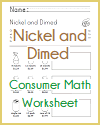Grade 4 Math - Measurement and Data | Student Handouts

 Fourth Grade Educational Materials > Fourth Grade Mathematics Materials > Measurement and Data
Solve problems involving measurement and conversion of measurements.
CCSS.MATH.CONTENT.4.MD.A.1: Know relative sizes of measurement units within one system of units including km, m, cm; kg, g; lb, oz.; l, ml; hr, min, sec. Within a single system of measurement, express measurements in a larger unit in terms of a smaller unit. Record measurement equivalents in a two-column table. For example, know that 1 ft is 12 times as long as 1 in. Express the length of a 4 ft snake as 48 in. Generate a conversion table for feet and inches listing the number pairs (1, 12), (2, 24), (3, 36), ...Metric Conversion Chart Consumer Math Worksheet
CCSS.MATH.CONTENT.4.MD.A.2: Use the four operations to solve word problems involving distances, intervals of time, liquid volumes, masses of objects, and money, including problems involving simple fractions or decimals, and problems that require expressing measurements given in a larger unit in terms of a smaller unit. Represent measurement quantities using diagrams such as number line diagrams that feature a measurement scale.
CCSS.MATH.CONTENT.4.MD.A.3: Apply the area and perimeter formulas for rectangles in real world and mathematical problems. For example, find the width of a rectangular room given the area of the flooring and the length, by viewing the area formula as a multiplication equation with an unknown factor.
Represent and interpret data.
CCSS.MATH.CONTENT.4.MD.B.4: Make a line plot to display a data set of measurements in fractions of a unit (1/2, 1/4, 1/8). Solve problems involving addition and subtraction of fractions by using information presented in line plots. For example, from a line plot find and interpret the difference in length between the longest and shortest specimens in an insect collection.
Geometric measurement: understand concepts of angle and measure angles.
CCSS.MATH.CONTENT.4.MD.C.5: Recognize angles as geometric shapes that are formed wherever two rays share a common endpoint, and understand concepts of angle measurement:
CCSS.MATH.CONTENT.4.MD.C.5.A: An angle is measured with reference to a circle with its center at the common endpoint of the rays, by considering the fraction of the circular arc between the points where the two rays intersect the circle. An angle that turns through 1/360 of a circle is called a "one-degree angle," and can be used to measure angles.
CCSS.MATH.CONTENT.4.MD.C.5.B: An angle that turns through n one-degree angles is said to have an angle measure of n degrees.
CCSS.MATH.CONTENT.4.MD.C.6: Measure angles in whole-number degrees using a protractor. Sketch angles of specified measure.
CCSS.MATH.CONTENT.4.MD.C.7: Recognize angle measure as additive. When an angle is decomposed into non-overlapping parts, the angle measure of the whole is the sum of the angle measures of the parts. Solve addition and subtraction problems to find unknown angles on a diagram in real world and mathematical problems, e.g., by using an equation with a symbol for the unknown angle measure.